# HBSE 10th Class Maths Important Questions Chapter 8 Introduction to Trigonometry

Haryana State Board HBSE 10th Class Maths Important Questions Chapter 8 Introduction to Trigonometry Important Questions and Answers.

## Haryana Board 10th Class Maths Important Questions Chapter 8 Introduction to Trigonometry

Question 1.
If tan2A = cot (A – 24°). Find A.
Solution :
We have,
tan 2A = coat (A – 24°)
tan 2A = tan (90° – (A – 24))
[∴ tan (90 – θ) = cotθ]
⇒ 2A = 90° – (A – 24°)
⇒ 2A = 90° – A + 24°
⇒ 2A + A = 114°
⇒ 3A = 114°
⇒ A = $$\frac {114}{3}$$ = 38°Question 2.
If cos3A = sin (A – 34°), where A is an acute angle, find the value of A.
Solution :
We have,
cos 3A = sin (A – 34°)
⇒ sin (90° – 3A) = sin (A – 34°)
[∴ sin (90 – θ) = cos θ]
⇒ 90° – 3A = A – 34°
⇒ 90° + 34° = A + 3A
⇒ 124° = 4A
⇒ A = $$\frac {124°}{4°}$$
⇒ A = 31° Hence proved

Question 3.
The value of (tan 1° tan 2° …. tan 89°) is equal to ?
Solution :
We have, tan 1° tan 2° tan 3° ….. tan 43° tan 44° tan 45° tan 46° tan 47° ….. tan 87° tan 88° tan 89° = tan 1° tan 2° tan 3° ….. tan 43° tan 44°
1. tan (90° – 44°) tan (90° – 43°) ….. tan (43° – 3°). tan (90° – 2°). tan (90° – 1°).
= tan 1° tan 2° tan 3° ….. tan 43° tan 44° : 1. cot 44° cot 43°….. cot 3° cot 2° cot 1
= tan 1° × $$\frac {1}{tan 1°}$$ . tan 2° × $$\frac {1}{tan 2°}$$ . tan 3° × $$\frac {1}{tan 3°}$$ ……… tan 43° × $$\frac {1}{tan 43°}$$ . tan 44° × $$\frac {1}{tan 44°}$$ . 1
= 1 × 1 × 1 × 1 × 1 × 1 = 1Question 4.
The value of (sin2θ + $$\frac{1}{1+\tan ^2 \theta}$$) = _____
Solution :
We have,
sin2θ + $$\frac{1}{1+\tan ^2 \theta}$$ = sin2θ + $$\frac{1}{\sec ^2 \theta}$$
[∴ 1 + tan2θ = sec2θ)
= sin2θ + cos2θ
= 1 Hence proved

Question 5.
(1 + tan2θ) (1 – sinθ) (1 + sinθ)
Solution :
(1 + tan2θ) (1 – sinθ) (1 + sinθ)
= sec2θ (1 – sin2θ)
= sec2θ × cos2θ
= $$\frac{1}{\cos ^2 \theta}$$ × cos2θ
= 1 Hence proved

Question 6.
Prove that : $$\frac{\tan A-\sin A}{\tan A+\tan A}=\frac{\sec A-1}{\sec A+1}$$
Solution :
We have,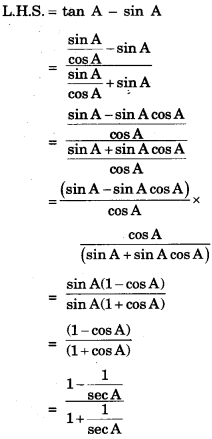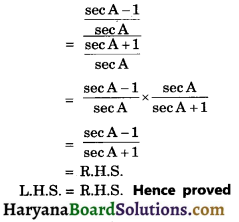Question 7.
Prove the following identify, where the angles involved are acute angles for which the expression is defined.
$$\frac{1+\cot ^2 \mathrm{~A}}{1+\tan ^2 \mathrm{~A}}$$ = ($$\frac{1-\cot A}{1-\tan A}$$)2
Solution :
We have,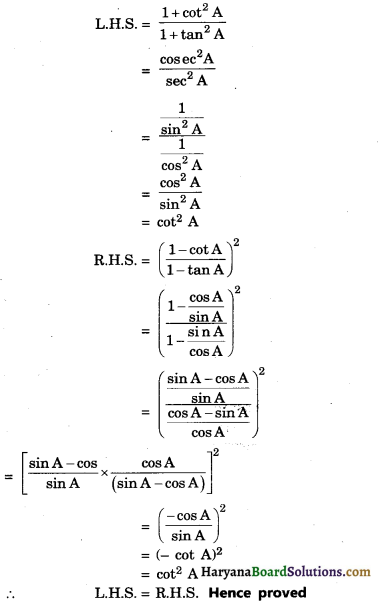Question 8.
Prove that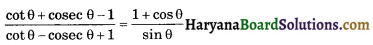Solution :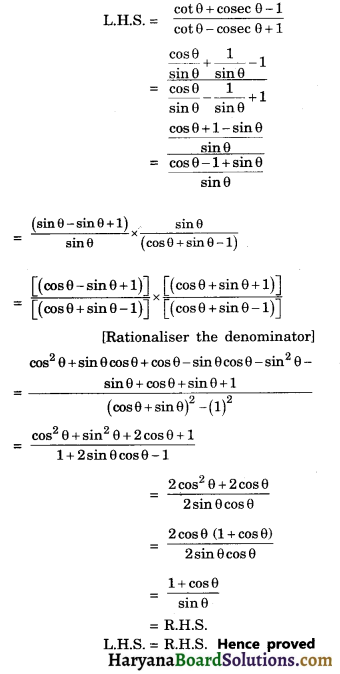Question 9.
Prove that :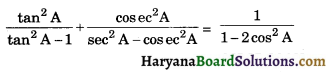Solution :
We have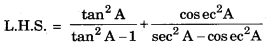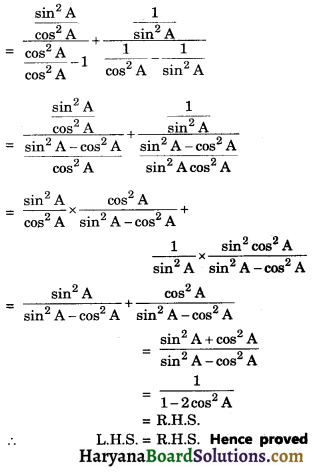Question 10.
Prove that: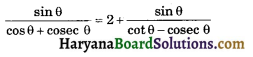Solution :
We have,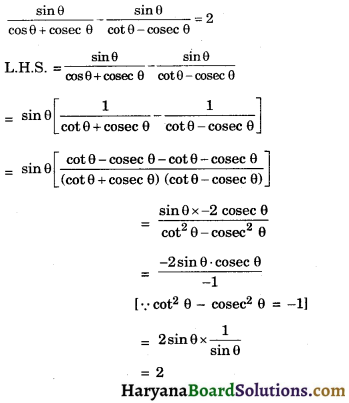= R.H.S
L.H.S. = R.H.S. Hence proved

Question 11.
Prove that: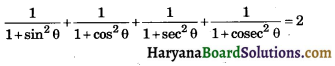Solution :
We have,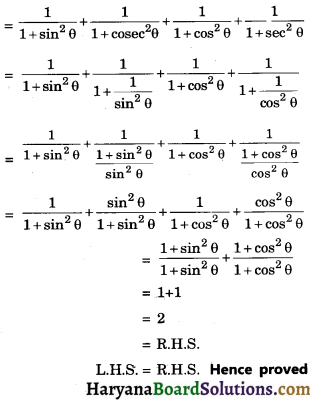Question 12.
If sin θ + cos θ = $$\sqrt{3}$$, then prove that tan θ + cot θ = 1
Solution :
We have,
sin θ + cos θ = $$\sqrt{3}$$
⇒ (sin θ + cos θ)2 = $$\sqrt{3}$$2
⇒ sin2θ + cos2θ + 2sin θ cos θ = 3
⇒ 1 + 2 sinθ cosθ = 3
⇒ sinθ cosθ = $$\frac {2}{2}$$ = 1 ………..(1)
Now L.H.S. = tanθ + cotθ
= $$\frac{\sin \theta}{\cos \theta}+\frac{\cos \theta}{\sin \theta}$$
= $$\frac{\sin ^2 \theta+\cos ^2 \theta}{\sin \theta \cos \theta}$$
= $$\frac {1}{1}$$[using equ. (1)]
= 1 = R.H.S.
L.H.S. = R.H.S. Hence provedQuestion 13.
If sin A + sinA2 = 1, then find the value of the expression cos2A + cost4A.
Solution :
We have, sin A + sin2A = 1
⇒ sin A = 1 – sin2A
⇒ sin A = cos2A
squaring both sides, we get
sin2A = cos4A
⇒ 1 – cos2A = cos4A
⇒ 1 = cos2A + cos4A
⇒ cos2A + cos4A = 1.
Hence proved

Fill in the Blanks

Question 1.
____ is the study of relationship between the sides and angles of a triangle.
Solution :
Trigonometry

Question 2.
An equation involving trignometric ratios of an angle is called a trignometeric.
Solution :
identityQuestion 3.
The word is derived from the Greek words tri (means three), gon (means sides), metron (means measure).
Solution :
Trigonometry

Question 4.
In a right triangle, aide opposite to right angle is known as _______ .
Solution :
hypotenuse

Question 5.
cot θ is the abbrevation used for ________ of angle θ.
Solution :
cotangent

Question 6.
The ratio of the sides of a triangle with respect to its acute angle are called trigonometric ____ of the angle.
Solution :
ratiosQuestion 7.
If ΔABC is right, right angled at C, then value of cos (A + B) is ______.
Solution :
0 (zero).

Multiple Choice Questions

Question 1.
If cos A = $$\frac {12}{13}$$, then cot A is :
(a) $$\frac {12}{5}$$
(b) $$\frac {13}{12}$$
(c) $$\frac {13}{5}$$
(d) $$\frac {5}{12}$$
Solution :
(a) $$\frac {12}{5}$$

Let us draw the triangle ABC in which ∠B = 90°
Then cos A = $$\frac{\mathrm{AB}}{\mathrm{AC}}=\frac{12}{13}$$
Let AB = 12k and AC = 13k
where k is a positive integer
By using Pythagoars Theorem, we have
AC2 = AB2 + BC2
(13k)2 = (12k)2 + BC2
BC2 = (13k)2 – (12k)2
BC2 = 169k2 – 144k2
BC2 = 25k2
$$\sqrt{\mathrm{BC}^2}$$ = 5k
cot A = $$\frac{\mathrm{AB}}{\mathrm{BC}}=\frac{12 k}{5 k}=\frac{12}{5}$$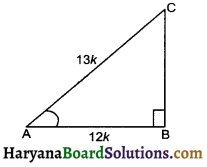Question 2.
The rod AC of TV disc antena is fixed at right angles to wall AB and a rod CD is supporting the disc as shown in given figure. If AC = 1.5 cm long and CD = 3m, then sec θ + cosec θ is :
(a) $$\frac {41}{12}$$
(b) $$\frac {41}{13}$$
(c) $$\frac {26}{12}$$
(d) $$\frac {15}{41}$$
Solution :
(b) $$\frac {41}{13}$$

The rod AC of TV disc antena is fixed at right angles to wall AB and rod CD is supporting the disc as shown. In right triangle ACD, we have
[By Phythogoras Theorem]
⇒ 32 = AD2 + 1.52
⇒ 32 – (1.5)2 = AD2
⇒ AD2 = 9 – 2.25
⇒ AD = $$\sqrt{6.75}$$
⇒ AD = 2.6 om (approx)
⇒ sec θ + cosec θ = $$\frac{C D}{A D}+\frac{C D}{A C}$$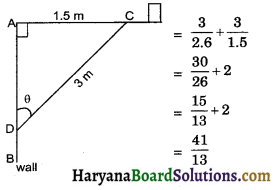Question 3.
If 4 tan θ = 3, then $\frac{4 \sin \theta-\cos \theta+1}{4 \sin \theta+\cos \theta+1}$ is :
(a) $$\frac {13}{5}$$
(b) $$\frac {5}{21}$$
(c) $$\frac {13}{21}$$
(d) $$\frac {12}{13}$$
Solution :
(c) $$\frac {13}{21}$$

We have, 4 tan θ = 3
⇒ tan θ = $$\frac{3}{4}=\frac{\mathrm{BC}}{\mathrm{AB}}$$
consider a triangle ABC in which ∠B = 90°
Let BC be 3k and AB be 4k wherek is positive integer.
In right triangle ABC, we have
AC2 = AB2 + BC2
= (4k)2 + (BC)2
= 16k2 + 9k2
= 25k2
AC = $$\sqrt{25 k^2}$$
= 5k
sin θ = $$\frac{3 k}{5 k}=\frac{3}{5}$$
and cos θ = $$\frac{4 k}{5 k}=\frac{4}{5}$$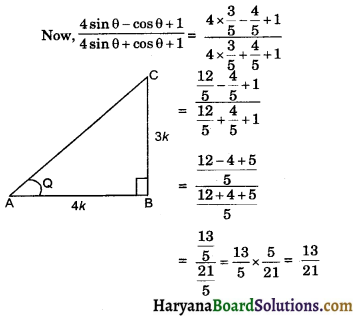Question 4.
If sin (A + 2B) = $$\frac{\sqrt{3}}{2}$$ and cos (A + 4B) = 0, A > B and A + 4B ≤ 90°, then A and B is :
(a) 30°, 45°
(b) 15°, 45°
(c) 60°, 15°
(d) 30°, 45°
Solution :
(d) 30°, 45°

We have,
sin (A + 2B) = $$\frac{\sqrt{3}}{2}$$
⇒ sin (A + 2B) = sin 60°
⇒ A + 2B = 60° ………(1)
And cos (A + 4B) = 0
⇒ Cos (A + 4B) = cos 90°
⇒ A + 4B = 90° ……(2)
Subtracting equation (2), from (1), we get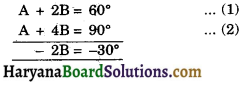⇒ B = $$\frac{\sqrt{-30}}{-2}$$ = 15°
Substituting the value of B in equ. (1), we get
A + 2 × 15 = 60°
⇒ A + 30° = 60°
⇒ A = 60 – 30 = 30°
Hence, A = 30° and B = 15°Question 5.
The value of sin 23° cos 67° + cos 23° sin 67° is:
Solution :
We have, sin 23° cos 67° + cos 23° sin 67°
= sin 23° cos (90° – 23°) + cos23° sin (90° – 23°)
= sin 23° sin 23° + cos 23° cos 23°
= sin2 23° + cos223°
= 1 [sin2θ + cos2θ = 1]

Question 6.
The value of sin 32° cos 58° + cos 32° sin 58° is:
(a) 0
(b) 1
(c) 2
(d) 3
Solution :
(b) 1

We have sin 32° cos 58° + cos 32° sin 58°
= sin 32° cos (90° – 32°) + cos 32° sin (98° – 32°)
= sin 32° sin 32° + cos 32° cos 32°
= sin232° + cos2 32°
= 1 [∴ sin2θ + cos2θ = 1]Question 7.
$$\frac{\cos 80^{\circ}}{\sin 10^{\circ}}$$ + cos 59° cosec 31° = :
(a) 0
(b) 1
(c) 2
(d) 3
Solution :
(c) 2

We have, $$\frac{\cos 80^{\circ}}{\sin 10^{\circ}}$$ + cos 59° cosec 31°
= $$\frac{\cos \left(90^{\circ}-10^{\circ}\right)}{\sin 10^{\circ}}$$ + cos 59° cosec (90° – 5°)
= $$\frac{\sin 10^{\circ}}{\sin 10^{\circ}}$$ + cos 59° sec 59°
= $$\frac{\sin 10^{\circ}}{\sin 10^{\circ}}+\frac{\cos 59^{\circ}}{\cos 59^{\circ}}$$
= 1 + 1 = 2